   shengsen@cnwire.org         +86-311-85293775
Galvanized Welded Wire Mesh specification calculation formula
You are here: » » » Galvanized Welded Wire Mesh specification calculation formula

# Galvanized Welded Wire Mesh specification calculation formula

Views:144     Author:Site Editor     Publish Time: 2018-12-27      Origin:Site Inquire

Galvanized Welded Wire Mesh specification calculation formula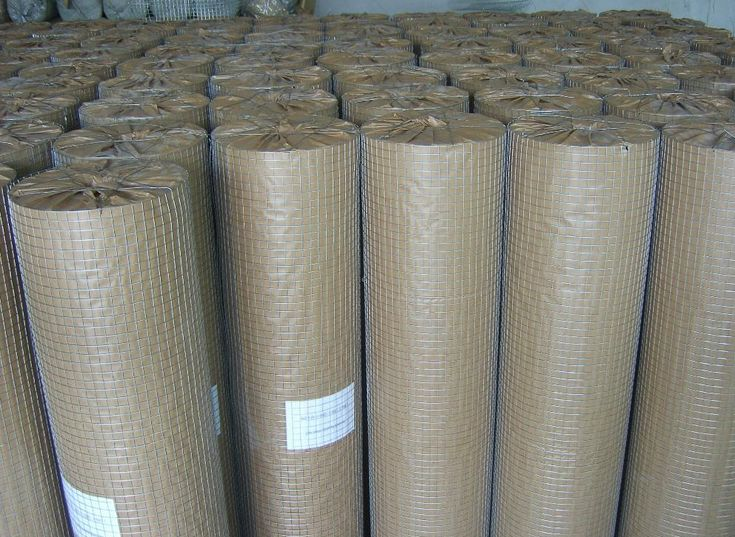1" weight calculation formula: wire diameter. × wire diameter × length x width ÷ 2 = kg

1"x1/2" weight calculation formula: wire diameter×wire diameter×length xwidth÷4x3=kg

1/2" weight calculation formula: wire diameter. × wire diameter. × length x width = kg

1/3" weight calculation formula: wire diameter. × wire diameter. × length x width x 3 x 0.48 = kg

1/4" weight calculation formula: wire diameter. × wire diameter. × length x width x 2 = kg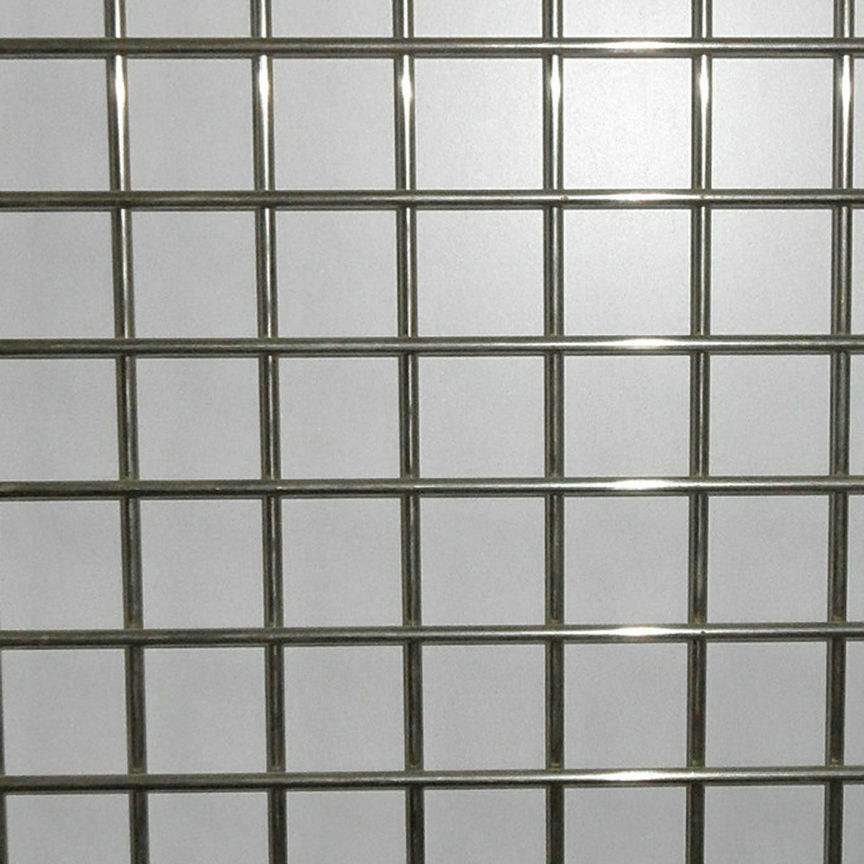3/4" weight calculation formula: wire diameter. × wire diameter. × length x width ÷ 2 x 1.33 = kg

3/8" weight calculation formula: wire diameter. × wire diameter. × length x width x 1.35 = kg

5/8" weight calculation formula: wire diameter.× wire diameter.× length x width ÷ 5 x 8÷2= kg

2"x4" weight calculation formula: wire diameter.× wire diameter.× length x width ÷ 2x 0.375= kg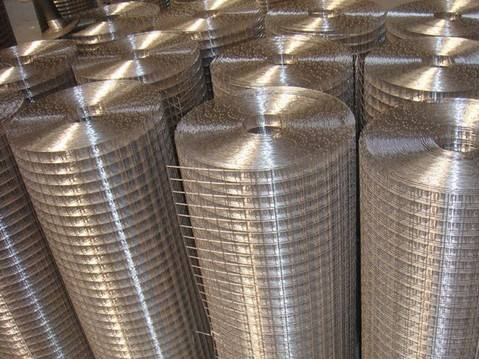1"x2" weight calculation formula: wire diameter.× wire diameter.× length x width ÷ 2x 0.75= kg

2"x3" weight calculation formula: wire diameter.× wire diameter.× length x width ÷ 2x 0.415= kg

2" x 2" weight calculation formula: wire diameter. × wire diameter. × length x width ÷ 4 = kg

3" x 3" weight calculation formula: wire diameter. × wire diameter. × length x width ÷ 6 = kg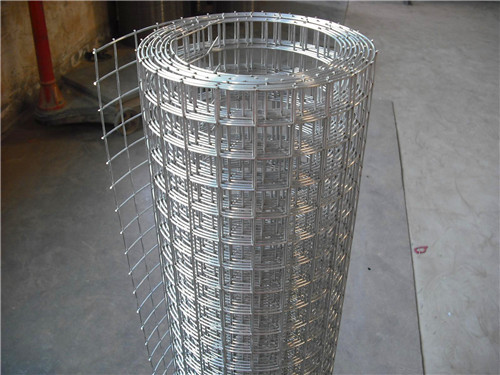4"x4" weight calculation formula: wire diameter.× wire diameter.× length x width ÷ 2x 0.25= kg

3"x4" weight calculation formula: wire diameter.× wire diameter.× length x width ÷ 2x 0.29= kg

Welded Wire Mesh winding diameter calculation method = wire diameter × length × 25.5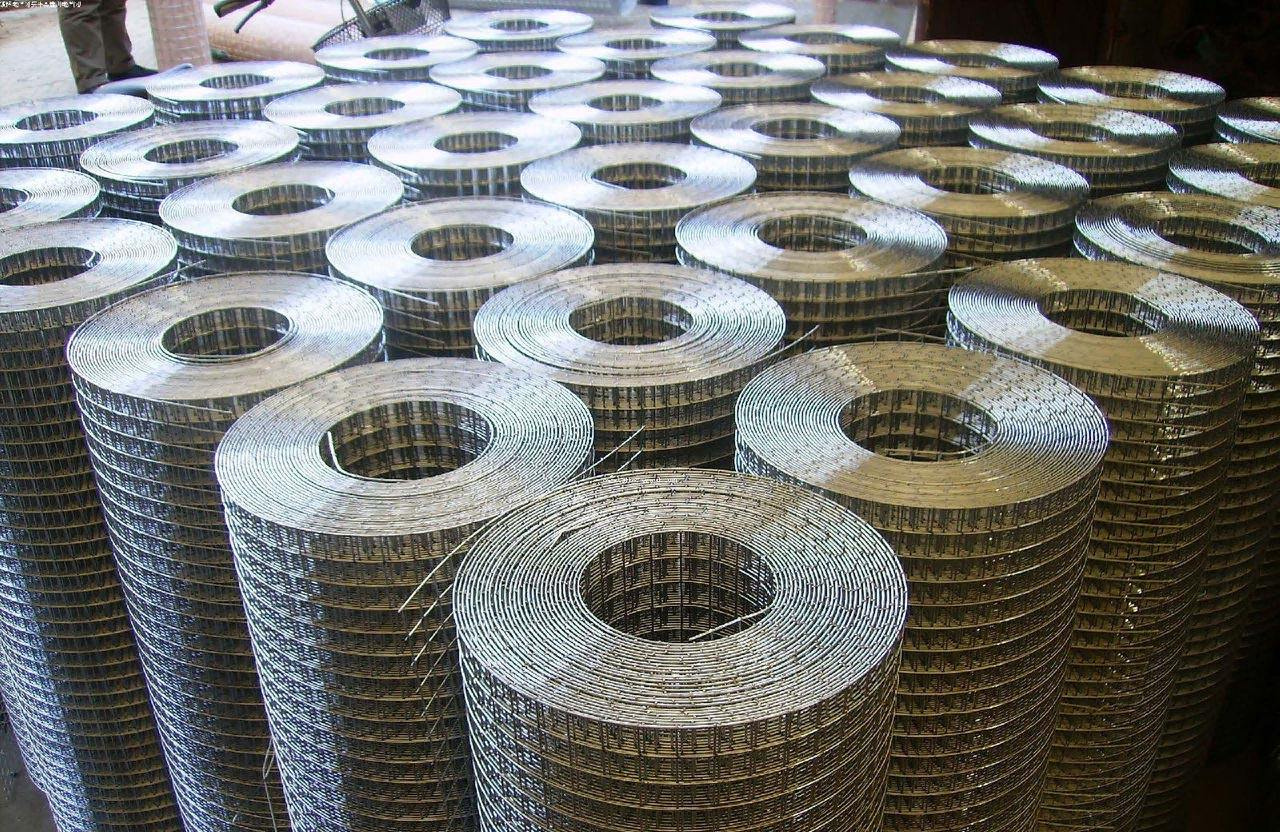Product Inquiry

## Recommend Products

This category is empty.

Room 1112, Baidu Building, No.38 Youyi South Street, Qiaoxi District, Shijiazhuang, China
E-MAIL
shengsen@cnwire.org
TEL
0086 311-85293775
0086 13731164509
HEBEI SHENGSEN METAL PRODUCTS CO.,LTD is located in Shijiazhuang city,the capital of Hebei Province,which is specialized in production and import-export of wire mesh products.   More >>
Room 1112, Baidu Building, No.38 Youyi South Street, Qiaoxi District, Shijiazhuang, China
Phone: 0086-13731164509
0086-18503279967

Tel: 0086-311-85293775